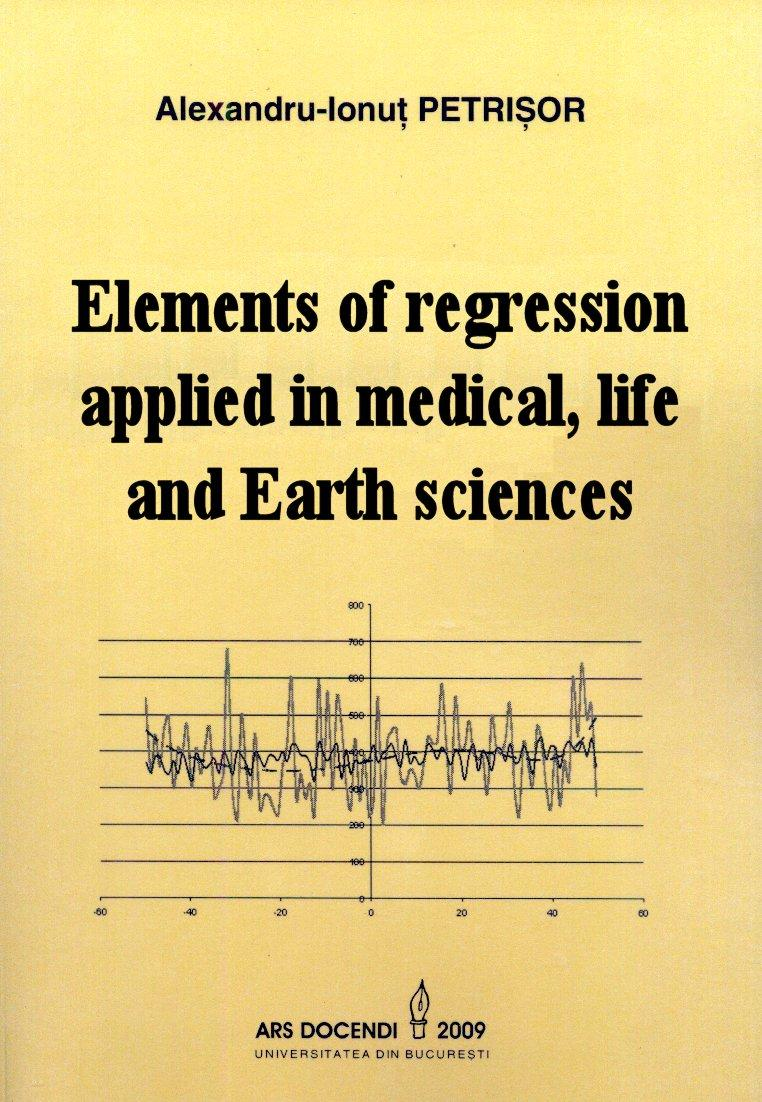# Elements of regression applied in medical, life and Earth sciencesAlexandru I. PETRIŞOR

109 pp., Ars Docendi Publishing House – University of Bucharest, April 2009, ISBN 978-973-558-416-0Born from the collaboration within the framework of research projects involving specialists in biology, ecology, geography, climatology, medicine, epidemiology, psychology, ethology, pedagogy and other disciplines, this work starts with the definition of regression (functional relationship between one or more correlated variables) in order to present the theoretical background of the statistical methodology used for the experimental design up to the interpretation of results, as well as concrete examples of applying the statistical instruments in the aforementioned fields. The book is addressed to specialists from these domains, especially to researchers, in order not to train them as statisticians, but prove that the scientific approach involves the appeal to a statistician even before starting the research, and facilitate their dialogue between these specialists and statisticians. Studies presented in about twenty peer reviewed papers published in Romanian and international journals, some indexed by Thompson Institute for Scientific Information, to which the author worked alone or as part of a research team, are analyzed with respect of phrasing research questions in statistical terms, using the appropriate statistical methodology, analyzing and interpreting the results. Apart from these, several “statistical jokes” are analyzed in order to prove that the use of some methods when data requirements are not met or inadequately could turn statistics into an instrument employed to prove anything, even the fact that a butterfly flapping its wings in Lausanne, Switzerland could be responsible for rainfalls in Paris.

Contents

Introduction

Chapter 1. Classification of variables

1.1. Distributions of variables

1.1.1. Binomial distribution

1.1.2. Poisson distribution

1.1.3. Normal (Gauss-Laplace) distribution

Chapter 2. Types of epidemiological studies. Advantages and disadvantages characteristic to each type

Chapter 3. Correlation and association

3.1. How do the wing flaps of a Brazil butterfly produce tornadoes in Texas?

3.2. Faith and criminality in America

3.3. Correlation, association and regression

Chapter 4. Types of regression

4.1. The t (Student) test

4.1.1. Nonparametric equivalent of the t test: Mann-Whitney-Wilcoxon test

4.2. One-way analysis of variance (ANOVA)

4.2.1. Generalities

4.2.2. Post-hoc (multiple) comparisons

4.2.3. Contrasts

4.2.4. Analysis of variance and the t test

4.2.5. Nonparametric equivalent of the analysis of variance: the Kruskal-Wallis test

4.3. Two-way analysis of variance

4.3.1. Nonparametric equivalent of the two-way analysis of variance: the Friedman test

4.4. Simple linear regression

4.5. Multiple linear regression

4.5.1. Special situations found in multiple linear regression

4.5.1.1. Modeling the interaction

4.5.1.2. Confounding

4.5.1.3. Model selection

4.6. Analysis of covariance (ANACOVA/ANCOVA)

4.7. Nonlinear regression

4.7.1. Polynomial regression

4.7.2. Logistic regression

4.7.2.1. Confounding in logistic regression

4.7.2.2. Model selection in logistic regression

4.7.3. Other nonlinear regression models

4.8. Cross-tabulation procedures. Analyses by strata

4.8.1. “Tetrapiloctomy” (literally: cutting the thread into four; over-splitting a problem)

4.8.2. Quantile regression

4.9. Analysis of spatial and temporal data

4.10. GLM (General/Generalized Linear Models)

4.10.1. Relationships between simple linear regression models with one independent variable and those with more independent variables

Chapter 5. Extensions of regression

References

Categories: Member publications